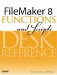# If()

Category: Logical

 Syntax: If ( test; result1; result2 )

Parameters:

testA logical expression that returns True (1) or False (0); result1The expression to evaluate if test is true; result2The expression to evaluate if test is false.

Data type returned: Text, Number, Date, Time, Timestamp, Container

Description:

The If() function returns one of two possible results depending on whether the test supplied as the first parameter is true or false. Result1 is returned if the test is true; Result2 is returned if the test is false.

The test parameter should be an expression that returns a numeric or Boolean result. For numeric results, zero and null are both considered false; all other values are considered true.

If the test contains multiple conditions separated by "and" or "or," FileMaker stops evaluating the conditions as soon as it can determine the overall truthfulness of the test. For instance, if the test parameter is IsEmpty(FieldA) and IsEmpty(FieldB), if Field A is not empty, theres no way that the entire expression could be true. FileMaker will not evaluate the other condition involving FieldB, and will return the false result.

You can nest If() statements within one another, but it is usually more efficient to use a Case() statement rather than an If() in such cases.

Examples:

``` If( DayOfWeek (Get (CurrentDate))) = 1 ;
"Its Sunday, no work!"; // true result
"Get back to work!" // false result
)

If ( myFlagField; graphicTrue; graphicFalse)
```

Looks for a true value (non-zero, non-blank) in myFlagField, and displays the correct graphic container.

``` If (not IsEmpty (Taxable) and TaxRate > 0;
Price + (Price * TaxRate);
Price
)
```FileMaker 8 Functions and Scripts Desk Reference
ISBN: 0789735113
EAN: 2147483647
Year: 2004
Pages: 352

Similar book on Amazon